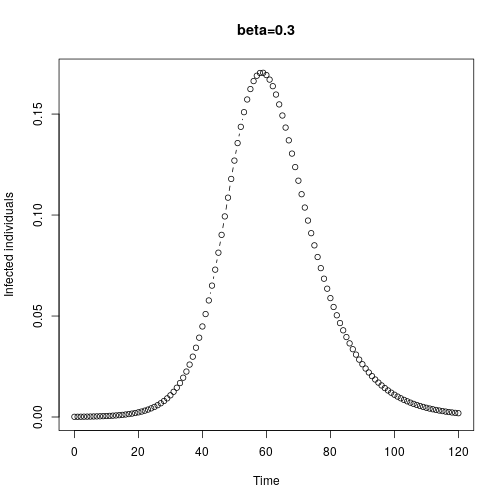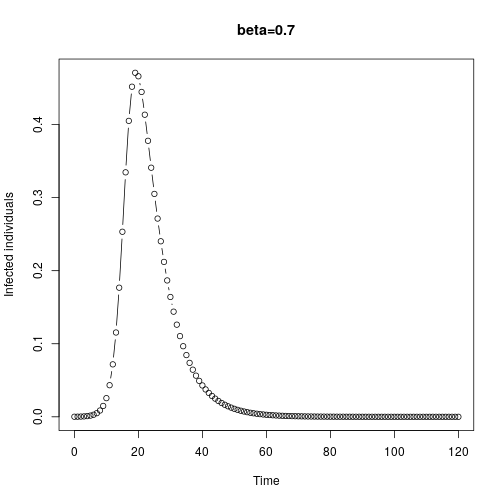# Basic reproduction number

John M. Drake

### Overview

Topics

• Basic reproduction number, $$R_0$$
• Invasion threshold
• Next generation method

### Epidemic trajactories differ according to parameters### Generalization

• Can we make general statements about epidemics without resorting to extensive numerical integration?
• Under what conditions will an infectious disease invade a system

### The invasion threshold

Assume an infectious individual arises in a Wholly Susceptible and otherwise Disease Free population

Initial conditions: $$X(0)=N-1 \approx N$$, $$Y(0)=1$$, and $$Z(0)=0$$

Invasion occurs only if $$dY/dt > 0$$

\begin{align} \frac{dY}{dt} = \beta X Y/N - \gamma Y &> 0 \\ Y(\beta X/N - \gamma) &>0 \\ X/N &> \gamma/\beta \end{align}

Since $$X \approx N$$, this is satisfied when $$1>\gamma / \beta$$, which is equivalent to $$\beta/\gamma > 1$$

Kermack & McKendrick (1927)

### Basic reproduction number

• For the $$SIR$$ model, the ration $$\beta/\gamma$$ gives te number of secondary cases that will be infected before the index case recovers
• This quantity is universally referred to as $$R_0$$ and called the Basic Reproduction Number or Basic Reproductive Ratio
• We can extend the concept of $$R_0$$ to other models with the following definition
• The basic reproduction number is the number of secondary cases generated a single typical infected case in an entirely suspectible population
• Hence, $$R_0$$ depends on both properties of the pathogen and properties of the population into which it is introduced